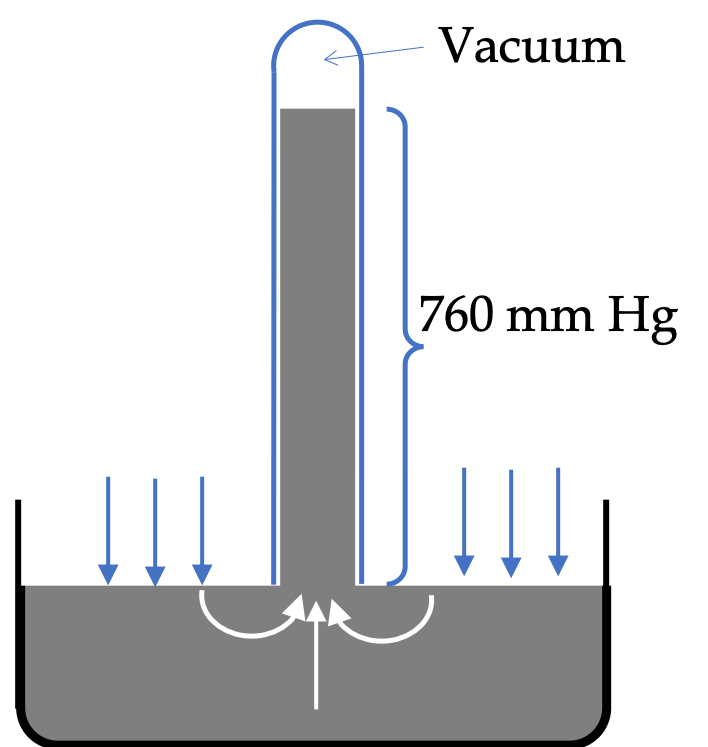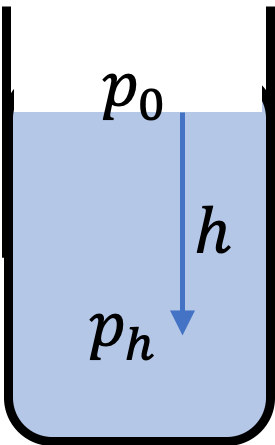## Section17.3Pressure in Fluid near Earth

### Subsection17.3.1Atmospheric Pressure

The atmosphere is invisible to the human eye, but it consists of gases and dust particles. The atmospheric pressure on your head may be assumed to come from the weight of all the gases above your head.

\begin{equation*} p_{\text{atm}} = \dfrac{W_{\text{air}}}{A}. \end{equation*}

Torricelli found a method by which he could measure this pressure. He used a tube with an opening at one end and closed at other. He filled the tube with mercury, inverted the tubed, and opened the open end inside a mercury bucket such that no air can enter the tube. The resulting apparatus is called a barometer.

Torricelli found that mercury in the tube empties out to a height of approximately 29.9 inches. In SI units that would be 760 mm. Torricelli correctly interpreted that the empty space above mercury in the tube was vacuum and that atmospheric pressure on the mercury in the bucket was pushing mercury into the tube.The weight of mercury in the tube with vacuum above it caused a downward force molecues at the neck which was balanced by the pressure in the mercury due to atmospheric pressure at the open surface of the bucket.

Thus, we can write the following equality.

\begin{equation*} \text{pressure at point level with outside} = \dfrac{\text{weight of mercury in tube}}{\text{area of tube}}. \end{equation*}

Let $\rho$ be the density of mercury, $A$ the area of cross-section of the tube, and $h$ the height of mercury in the tube (which is 760 mm).

\begin{equation*} \text{pressure at point level with outside} = \dfrac{ \rho A h g}{A} = \rho g h. \end{equation*}

Putting numerical values, this gives the following for the atmospheric pressure in SI units.

\begin{equation*} 13,690 \text{ kg/m}^3 \times 9.81\text{ m/s}^2 \times 0.760\text{ m} = 102,067.1\text{ Pa}. \end{equation*}

A little history

Originally, when the same experiment was done with water in the inverted tube, water was always pushed to the end in the inverted tube for all the lengths of the tubes tried. As a matter of fact atmospheric pressure can support approximately 34 feet of water column, which was too long a tube. It was interpreted to indicate that nature “abhors” vacuum. Torricelli was Galileo's secretary in the last three months of latter's life. Galileo apparently suggested to Torricelli to try mercury since mercury was a much denser substance and a smaller tube might be sufficient.

### Subsection17.3.2Pressure in a Fluid with Surface Open to Atmosphere

Pressure in a fluid varies with depth due to the different weight of fluid above that level. For instance, at the surface of ocean, we have pressure from the air above that point, which is the atmospheric pressure. We will denoted atmospheric pressure $p_0\text{.}$

At depth $h$ in the ocean, we have both the weight of the air and the weight of water of height $h$ above that point. With $\rho_w$ for the density of water, we will get the following for the pressure at depth $h$ in the ocean to be

\begin{equation*} p_h = p_{0} + \dfrac{\rho_w A h g}{A}. \end{equation*}

This simplifies to

\begin{equation} p_h = p_{0} + \rho_w g h.\tag{17.3.1} \end{equation}This formula will apply also to other fluids with surface open to atmosphere. We just need to replace the density by the density of that fluid. Therefore, let us write the formula for pressure at depth $h$ in any fluid with density $\rho\text{.}$

\begin{equation} p_h = p_{0} + \rho g h.\tag{17.3.2} \end{equation}

### Subsection17.3.3Atmospheric Pressure Above Sea Level

Air thins out with altitude with both temperature and pressure varying with height. A simple model of atmosphere gives the following for “atmospheric pressure” at altutude $h$ above the sea level.

\begin{equation} p_h = p_{0}\,e^{-\alpha h},\tag{17.3.3} \end{equation}

where $p_0$ is the atmosphereic pressure at the sea level and constant $\alpha$ is the rate at which pressure drops as you go up in altitude with the following approximate value.

\begin{equation*} \alpha = \dfrac{1}{9,000\text{ m}}. \end{equation*}

Constant $\alpha$ can be related to mass of an air molecule $m$ and temperature in kelvin scale $T\text{.}$

\begin{equation*} \alpha = \dfrac{mg}{k_B T}. \end{equation*}

Say, air is all nitrogen molecules. Then, you would have at room temperature of $27^\circ\text{C}\text{,}$

\begin{align*} m \amp= \dfrac{28\times 10^{-3}}{6.02\times 10^{23}} = 4.65\times 10^{-26}\text{ kg},\\ T \amp= 27+273 = 300\text{ K},\\ k_B \amp = 1.38 \times 10^{-23} \text{ m}^2\text{kg}\text{ s}^{-2}\text{K}^{-1},\\ g \amp = 9.81\text{ m/s}^2. \end{align*}

This should give approximately the value of $\alpha$ quoted above.

Depth in a pond varies from 1 meter to 5 meters. What is the range of pressure at the bottom across the pool?

Hint

Use $p_h = p_0 + \rho g h\text{.}$

$111,000\text{ Pa}$ to $150,000\text{ Pa}\text{.}$

Solution

The pressure in a static fluid with open surface is given by

\begin{equation*} p_h = p_0 + \rho g h. \end{equation*}

Let us denote by $p_1$ the pressure at 1 meter depth and by $p_5$ the pressure at 5 meter depth.

\begin{align*} p_1 \amp = 1.013\times 10^5\text{ Pa} + 1000\text{ kg/m}^3 \times 9.81\text{ m/s}^2 \times 1\text{ m}, \\ \amp = 111,000\textrm{ Pa}, \\ p_5 \amp = 1.013\times 10^5\text{ Pa} + 1000\text{ kg/m}^3 \times 9.81\text{ m/s}^2 \times 5\text{ m},\\ \amp = 150,000\textrm{ Pa}. \end{align*}

Note: $p_5$ is not $5\times p_1\text{.}$

Acceleration due to gravity at moon is $\dfrac{g}{6}\text{.}$ What is the pressure at a depth of $2\text{ m}$ in a tank of water at moon?

Hint

Use the fact that there is no atmosphere at moon.

$3270\text{ Pa}\text{.}$

Solution

Since there is no atmosphere, the pressure will come from weight of the fluid particles alone.

\begin{align*} p \amp= \rho g_{\text{moon}} h\\ \amp= 1000\ \text{kg/m}^3 \times \dfrac{9.81\ \text{m/s}^2}{6} \times 2\ \text{m} = 3270\ \text{Pa}. \end{align*}

What is the difference of pressure at the top and bottom of a $10\text{-m}$ tall sealed container filled with air with density $1.3\text{ kg/m}^3\text{?}$

Hint

Only $\rho g h\text{.}$

$110\text{ Pa}\text{.}$

Solution

Since the container is sealed, it does not matter where the container is. The formula for the difference in pressure over a height difference $\Delta h$ will be

\begin{align*} \Delta p \amp = \rho g \Delta h, \\ \amp = 1.3\text{ kg/m}^3 \times 9.81\text{ m/s}^2 \times 10\text{ m},\\ \amp = 110\text{ Pa}. \end{align*}

What is the air pressure at an altitude of $40,000\text{ feet}\text{?}$

Data: $p_0 = 1.013\times 10^{5}\text{ Pa}\text{.}$

Hint

Use $p_h = p_0 \exp(-\alpha y)\text{.}$

$1.004\times 10^5\text{ Pa}\text{.}$

Solution

Let us first convert $40,000\text{ feet}$ to meters first.

\begin{equation*} 40,000\ \text{ft} = \frac{40,000\ \text{ft}}{12\ \text{in/ft}} \times 2.54\times 10^{-2}\ \text{m/in} = 84.7\ \text{m}. \end{equation*}

Now we plug this value of the height in $p_h = p_0 \exp(-\alpha y)$ with $\alpha = 1/9,000\text{ m}$ to obtain

\begin{equation*} p = 1.004\times 10^{5}\ \text{Pa}. \end{equation*}

### Subsection17.3.4(Calculus) Variation of Atmospheric Pressure with Height

If the density of air didn't vary with height, the pressure will vary with height in a linear way. This can be seen as follows. Suppose the atmosphere goes up to a height H. Since, there would be nothing above it, the pressure will be zero there. If we want pressure at height $h$ from the sea level, we will just use the weight of air above $h\text{,}$ which will be the air of a column of height $H-h\text{.}$ Thus, the pressure at height $h$ would be

\begin{equation*} p_h = \rho_a g (H-h). \end{equation*}

But, this is wrong! We will use a result from thermodynamics to make our point. In a gas, we can use ideal gas law, $pV=Nk_BT\text{,}$ which can be also written as

\begin{equation*} \rho = \dfrac{m}{k_B T}\, p, \end{equation*}

where $m$ is mass a gas molecule, which we can approximate to the mass of a nitorgen, $N_2 \text{,}$ molecule, $k_B$ a universal constant, called the Boltzmann constant, and $T$ the temperature in kelvin. Let us use a $y$ axis pointed up with origin at the sea level.

Then, at height, now given by coordinate $y\text{,}$ the density and pressure are related by

\begin{equation} \rho(y) = \dfrac{m}{k_B T}\, p(y),\label{eq-ideal-gas-pressure-density}\tag{17.3.4} \end{equation}

But, $p(y)$ is just the pressure from all the air above $y\text{.}$

\begin{equation*} p(y) = \dfrac{1}{A}\, g\, \int_{y}^{\infty} \rho(y) A dy, \end{equation*}

from which we get the following differential relation.

\begin{equation*} \dfrac{dp}{dy} = -g \rho(y), \end{equation*}

which can be rewritten with the help of Eq. (17.3.4) as

\begin{equation} \dfrac{dp}{dy} = -\alpha p,\label{eq-pressure-varying-with-y-diff-eq}\tag{17.3.5} \end{equation}

where

\begin{equation} \alpha = \dfrac{mg}{k_B T}.\tag{17.3.6} \end{equation}

Eq. (17.3.5) can be rearranged and then integrated from $y=0$ to $y=y\text{.}$

\begin{equation*} \int_{p_0}^{p(y)}\dfrac{1}{p} dp = -\int_0^y \alpha dy. \end{equation*}

This gives

\begin{equation*} \ln \dfrac{p(y)}{p_0} = -\alpha y, \end{equation*}

which is solve for $p\text{.}$

\begin{equation} p(y) = p_0\, e^{-\alpha y}.\tag{17.3.7} \end{equation}

This shows that the atmospheric pressure decreases exponentially with height $y \text{,}$ and not linearly. The pressure drops by a factor of $\frac{1}{e}$ when height from the sea level is $\dfrac{1}{\alpha}\text{.}$ Based on this we get a physical interpretation for $\alpha \text{:}$ constant $\dfrac{1}{\alpha}$ is a length scale that characterizes how pressure varies with height.

An approximate value of $\alpha$ can be obtained by using mass of a nitrogen molecule as a proxy for an air molecule. At temperature $27^{\circ}\text{C}$ or $300\text{K}\text{,}$ we find

\begin{equation} \alpha=-\frac{mg}{k_B T} = \frac{1}{9,000\ \text{m}}. \tag{17.3.8} \end{equation}

Therefore, for every 9,000 meters, the air pressure drops by a factor $\dfrac{1}{e}$ or approximately one-third of its value. Of course, this gives us only a rough estimate of the physical situation since we have assumed both the temperature and $g$ constant over such great distances from the Earth, neither of which is correct in reality.

Solve $\dfrac{dp}{dy} = - 3 p^2\text{,}$ given $p (0) = 1\text{ atm}\text{.}$

Hint

Integrate the differential equation.

$p(y) = \dfrac{1}{1+3y}\text{.}$

Solution

This is a straight forward math problem.

\begin{equation*} \dfrac{dp}{dy} = - 3 p^2\ \ \Longrightarrow\ \ \dfrac{dp}{p^2} = - 3 dy. \end{equation*}

Now integrate both sides to obtain

\begin{equation*} \dfrac{1}{p} = 3 y +C, \end{equation*}

where $C$ is the constant of integration. The condition at $y=0$ gives

\begin{equation*} 1 = 0 + C. \end{equation*}

Therefore,

\begin{equation*} \dfrac{1}{p} = 3 y +1, \end{equation*}

which we can rewrite as

\begin{equation*} p(y) = \frac{1}{1+ 3y}. \end{equation*}

Solve $\dfrac{dp}{dy} = - 3 \exp{(-2y)}\text{,}$ given $p(0) = 1\text{ atm}\text{.}$

Hint

Integrate the differential equation.

$p(y) = \dfrac{3}{2} \exp{(-2y)} -\dfrac{1}{2}.$

Solution

We write the given equation as

\begin{equation*} dp = - 3 \exp{(-2y)} dy, \end{equation*}

which can be integrated to obtain

\begin{equation*} p = \dfrac{3}{2} \exp{(-2y)} + C. \end{equation*}

Now, we fit the condition at $y=0$.

\begin{equation*} 1 = \dfrac{3}{2} +C\ \ \Longrightarrow\ \ C = -\dfrac{1}{2}. \end{equation*}

Therefore

\begin{equation*} p (y) = \dfrac{3}{2} \exp{(-2y)} -\dfrac{1}{2}. \end{equation*}